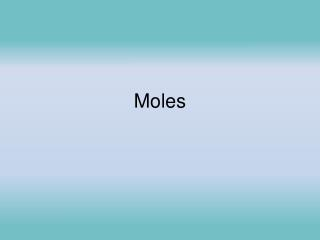DownloadDownload PresentationMoles

# Moles

Télécharger la présentation## Moles

- - - - - - - - - - - - - - - - - - - - - - - - - - - E N D - - - - - - - - - - - - - - - - - - - - - - - - - - -
##### Presentation Transcript

1. Moles

2. The Mole • This is a unit of measurement.

3. Moles • 1 mole = 6.02x 1023 particles

4. Find amount of particles and weight 1 mole of copper atoms= 1 mole of NaCl Formula units= 1 mole of water molecular units=

5. 1 mole of copper atoms= 6.02x1023 atoms= 63.5g 1 mole of NaCl Formula units= 6.02x1023 fu 58.5g 1 mole of water molecular units= 6.02x1023 mc= 18g

6. Avagadro’s number = 6.02x1023 particles

7. In a lab 0.36mol Al are needed. How many particles is that? What type of particle is this?

8. In a lab 0.36mol Al are needed. How many particles is that? What type of particle is this? 0.36mol X6.02x1023 particles = 2.1672 x1023 1 mol Al 2.2 x1023 atoms

9. How many moles of Na2CO3 contain 7.9x1024 f. units?

10. How many moles of Na2CO3 contain 7.9x1024 f. units? 7.9x1024 f.units x 1 mole 6.02x1023 f.units 13.1229 13 moles

11. Mole to mass problems • What is the molar mass of these substances? Pb H2O  Cu  Al  NaCl 

12. If you had 3.5 moles of Pb what is the mass

13. If you had 3.5 moles of Pb what is the mass 3.5 mol Pb x 207.2g Pb 725.2g 1 mol Pb 730g

14. If I had 125.1 g H2O how many moles is that?

15. If I had 125.1 g H2O how many moles is that? 125.1g H2O x 1mol H2O 6.950mol 18.0g H2O 6.950mol

16. In groups You have 6.0 moles of oxygen gas, what is the mass?

17. You have 6.0 moles of Oxygen gas, what is the mass? 6.0moles O x 32.0g O 192 grams 1mol O =200g

18. In groups The mass of a sample of ammonia is 232grams. How many moles are in the sample?

19. The mass of a sample of ammonia is 232grams. How many moles are in the sample? 232g NH3 x 1 mol 13.647mol 17.0 g 13.6 mol

20. Volume 1 mole of any gas at STP has a volume of 22.4L STP=Standard Temperature and Pressure 0o C and 1 atm 1 mol gas = 22.4L

21. If you have 3.5 mol of O2 gas what is the volume?

22. If you have 3.5 mol of O2 gas what is the volume? 3.5 mol O2 x 22.4 L O2 1 mol O2 78.4 L 78L

23. If a gas has a volume of 10.0L, how many moles is that?

24. If a gas has a volume of 10.0L, how many moles is that? 10.0 L x 1 mol 22.4 L =0.4462 mol 0.446 mol

25. What is the unit/ type of particle/ amount for Representative particles for each of the following: Matter: Element Ionic cmpd Molecular cmpd R. Part. Type: _______ __________ ____________ Unit: _______ __________ ____________ Amount Of Part. _______ __________ ____________

26. What is the unit/ type of particle/ amount for Representative particles for each of the following: Matter: Element Ionic cmpd Molecular cmpd R. Part. Type: atoms formula units molecules Unit: atoms formula units molecules Amount Of Part. 6.02x1023 atoms 6.02x1023 formula units 6.02x1023 molecules

27. SOLVE Convert 0.50 moles of ammonia vapor to liters. How many particles are in 0.35 mol of CaCl2 (what type of particles will we have?)

28. Convert 0.50 moles of ammonia vapor to liters. 0.50 mol x 22.4 L 1mol =11.2L = 11L

29. How many particles are in 0.35 mol of CaCl2 (what type of particles will we have?) 0.35 mol x 6.02x1023f.units 1mol =2.107x1023 f.units = 2.1x1023 f.units

30. Mole to Mole Ratio’s Balance: Mg + O2 MgO N2H4 + N2O4  N2 + H2O

31. 2 Mg + O2 2 MgO 2 N2H4 + N2O4  3 N2 + 4 H2O

32. Coefficients represent the number of moles for each element. This gives us a ratio in the rnx. 2 Mg + O2 2 MgO ____Mg:____MgO ____Mg:____O2 ____O2: ____MgO

33. 2 Mg + O2 2 MgO __2__Mg:__2__MgO ___2_Mg:__1__O2 __1__O2: __2__MgO

34. 2 Mg + O2 2 MgO If 3.5 moles of Mg react __(#1)___moles of O2 to produce __(#2)__ mole of MgO #1 3.5 mol Mg x 1 mol O2 =1.75 mol O2 2 mol Mg = 1.8 mol O2 #2 3.5 mol Mg x 2 mol MgO = 3.5 mol MgO 2 mol Mg

35. If 3.5 moles of Mg react __1.8___moles of O2 to produce _3.5___ mole of MgO

36. 2 N2H4 + N2O4  3 N2 + 4 H2O 3.75 moles of N2O4 Find the other amount of moles needed. If 3.75 mol N2O4 rnx with ____ mol N2H4 they will produce ___ mol N2 and ___ mol H2O.

37. 2 N2H4 + N2O4  3 N2 + 4 H2O 3.75 mol N2O4 x 2 mol N2H4 1 mol N2O4 =7.50 mol N2H4 3.75 mol N2O4 x 3 mol N2 1 mol N2O4 =11.25 mol N2 =11.3 mol N2 3.75 mol N2O4 x 4 mol H2O 1 mol N2O4 =15.0 mol H2O